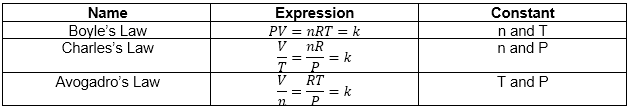# Problem: In an air-conditioned room at 19.0 °C, a spherical balloon had the diameter of 50.0 cm. When taken outside on a hot summer day, the balloon expanded to 51.0 cm in diameter. What was the temperature outside in degrees Celsius? Assume that the balloon is a perfect sphere and that the pressure and number of moles of air molecules remains the same.Express your answer with the appropriate units.The ideal gas law (PV = nRT) describes the relationship among pressure P, volume V, temperature T, and molar amount n. When some of these variables are constant, the ideal gas law can be rearranged in different ways to take the following forms where k is a constant:

###### FREE Expert Solution
81% (266 ratings)View Complete Written Solution
###### Problem Details

In an air-conditioned room at 19.0 °C, a spherical balloon had the diameter of 50.0 cm. When taken outside on a hot summer day, the balloon expanded to 51.0 cm in diameter. What was the temperature outside in degrees Celsius? Assume that the balloon is a perfect sphere and that the pressure and number of moles of air molecules remains the same.

The ideal gas law (PV = nRT) describes the relationship among pressure P, volume V, temperature T, and molar amount n. When some of these variables are constant, the ideal gas law can be rearranged in different ways to take the following forms where k is a constant: